sian.mat

•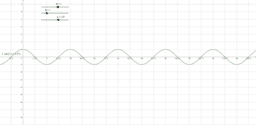Rsin(Nx+a)

Activity

sian.mat

•Wave Equation for PowerPoint Example

Activity

sian.mat

•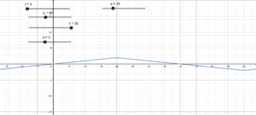Wave Equation

Activity

sian.mat

•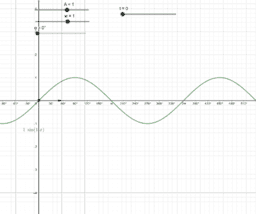Vector representation of phasors

Activity

sian.mat

•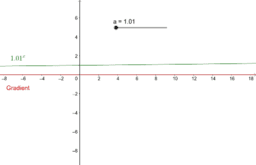Differentiation of e^x

Activity

sian.mat

•Fourier Demonstration

Activity

sian.mat

•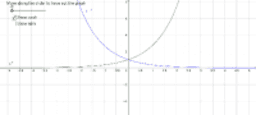Hyperbolic Graphs

Activity

sian.mat

•Activity

sian.mat

•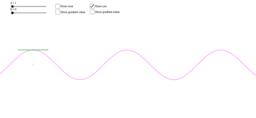Demonstration of sin and cos differential

Activity

sian.mat

•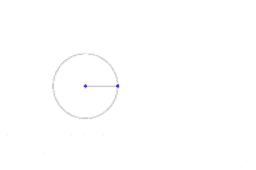Activity

sian.mat

•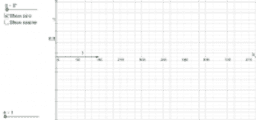sine and cos

Activity

sian.mat

•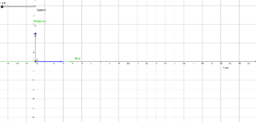AC current as phasor

Activity

sian.mat

•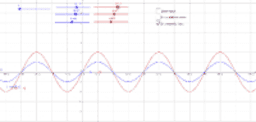Activity

sian.mat

•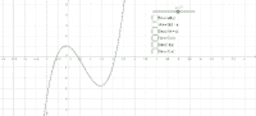Graph Transformations

Activity

sian.mat

•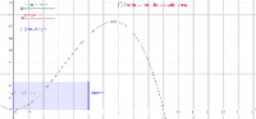Comparing Integration to Area Under Graph

Activity

sian.mat

•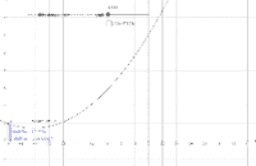Demonstration of how integration gives us area under a curve

Activity

sian.mat

•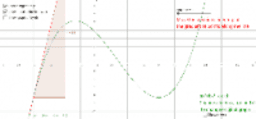Activity

sian.mat

•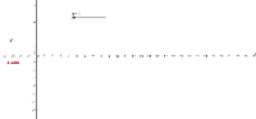Differentiation of e^x

Activity

sian.mat

•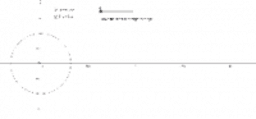Activity

sian.mat